I got interested in Homography a few days back since it was needed for my research. So I though why not do a simple tutorial showing how to use OpenCV to generate a basic panorama.

But! for that you need to understand what a Homography is. Go through section 2.1 and 2.2 of this paper (Malis): https://hal.archives-ouvertes.fr/file/index/docid/174739/filename/RR-6303.pdf

To summarize it: Suppose we have two images of the same planar object with some overlap between them. Let p1 be the pixel coordinates [p1x, p1y, 1] of a point in 1st image and p2 be the pixel coordinates [p2x, p2y, 1] of a point in the 2nd image. Let us call the 1st image as the destination image and the 2nd image as the source image. The Homography 1H2 relates every pixel coordinate in the 2nd image to a pixel coordinate in the 1st image.

p1 = 1H2 * p2


If you think of the 1st image in a bigger canvas where everything surrounding it is black (since we don’t have data around it), the related pixel coordinates from the second image will bring in more data and will stick around those black part! Think about this :)

Anyways, I wanted to get started with coding this Homography retrieval using OpenCV in C++. To find the homography between any two source and destination images, we need to have at-lest 4 point to point correspondences. Getting these correspondences are easy if you use some kind of feature detector and match them via their descriptors. Examples of some feature detectors are SIFT, SURF, ORB etc etc. We will stick to SIFT here. They are a bit slow compared to other options but are most accurate.

(1.) So we start with 2 images. Image 1 is the destination and Image 2 is the source. Both have some overlap between them.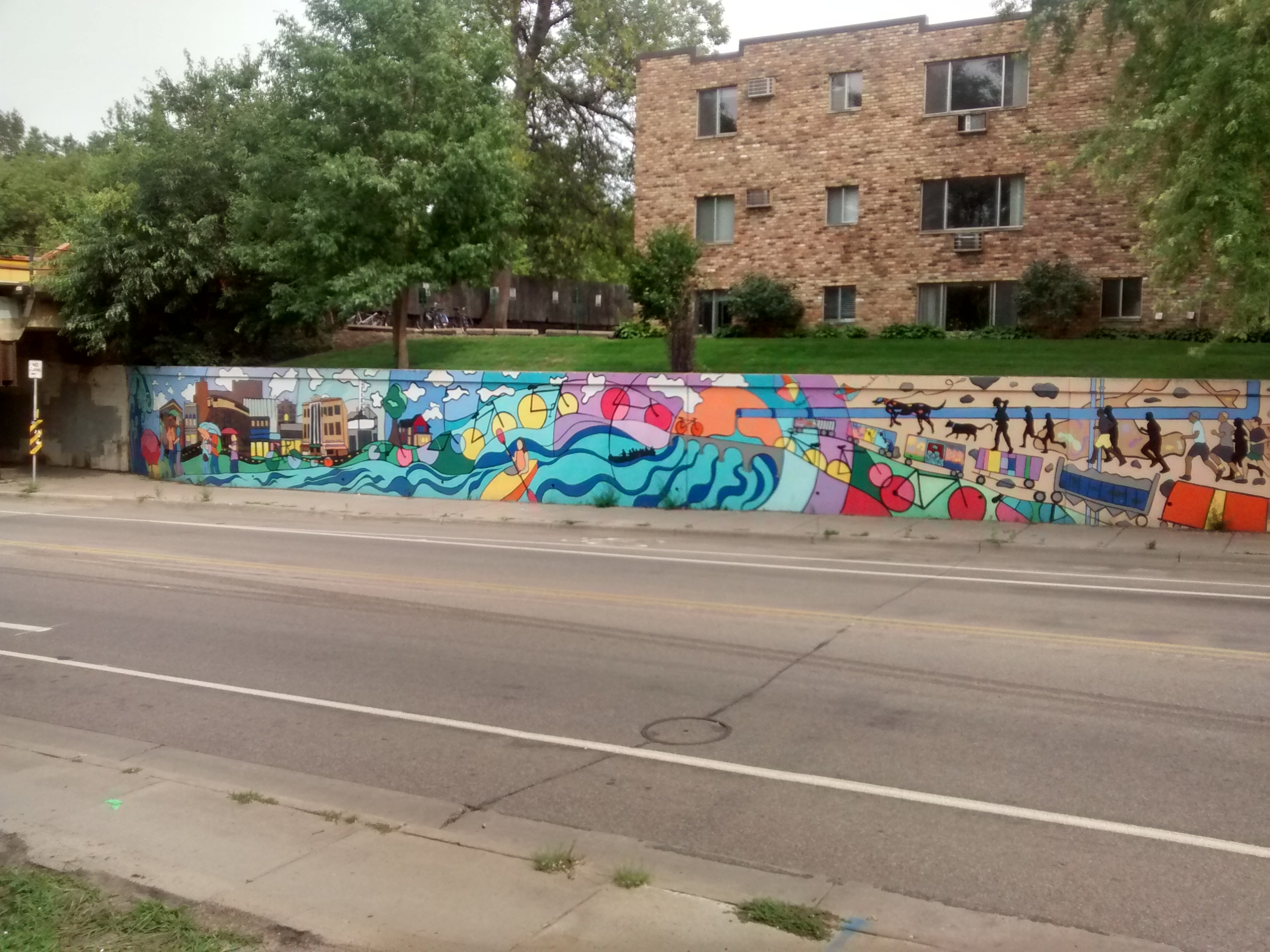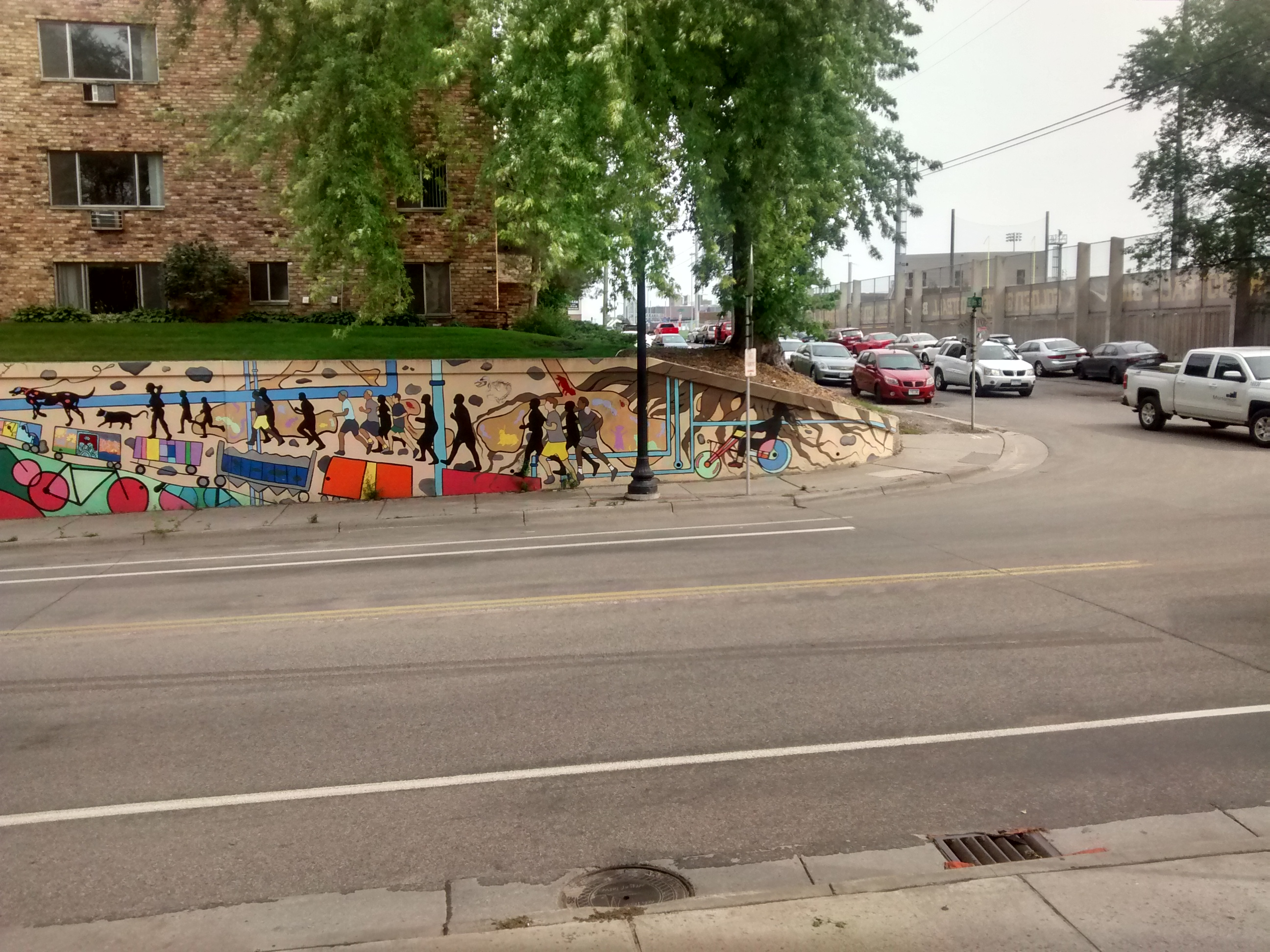(2.) As I mentioned previously, we need to think of the destination image on a bigger canvas if we want to stick the pixels from the source image around it. Let’s do that.

int offsetx = 800;
int offsety = 1000;
Mat trans_mat = (Mat_<double>(2, 3) << 1, 0, offsetx, 0, 1, offsety);
warpAffine(im1, im1, trans_mat, Size(3 * im1.cols, 3 * im1.rows));


The result: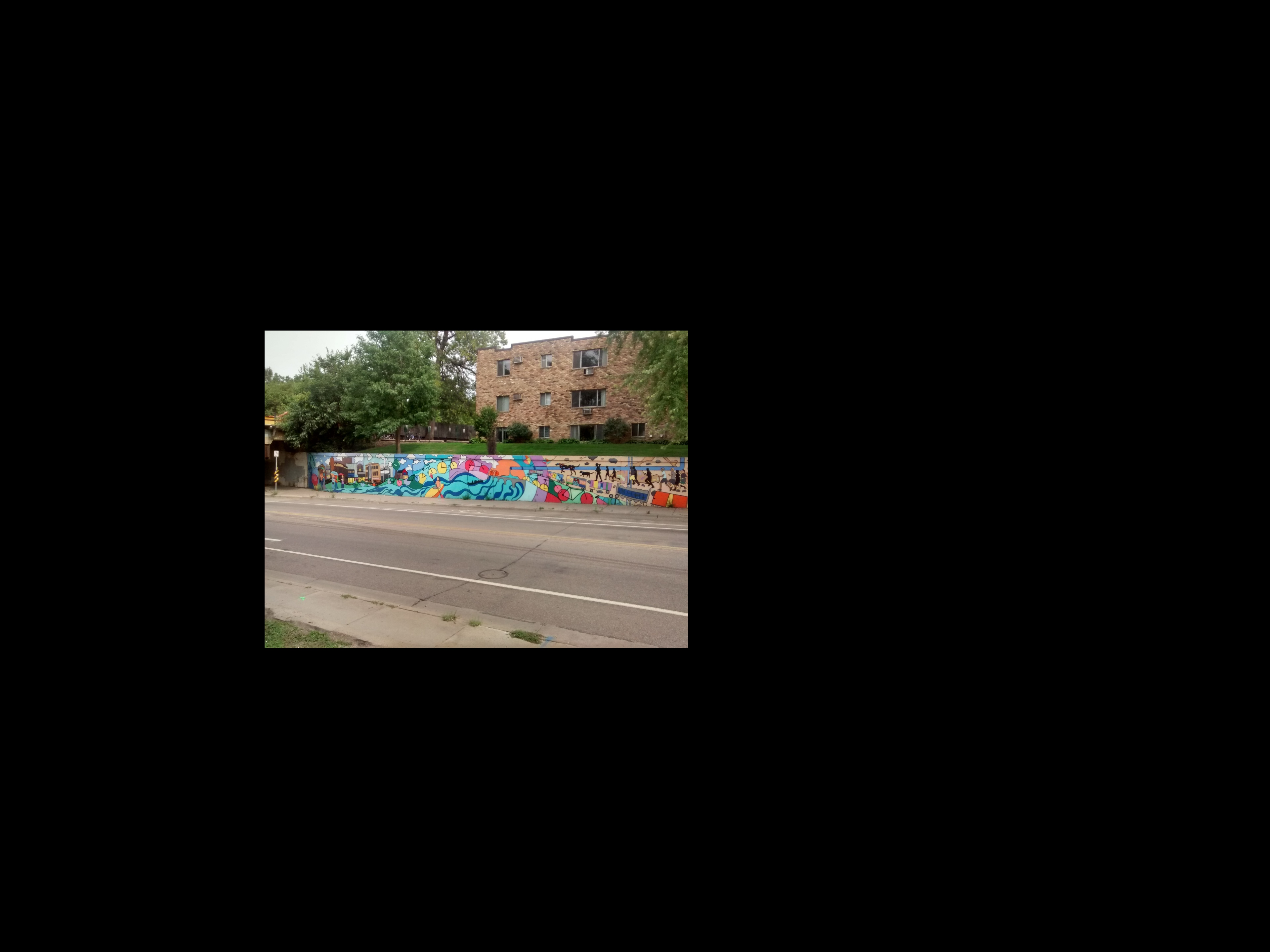(3.) Now we will find the SIFT feature matches between the current bigger canvased image: im_1 and the other image im_2.

cv::Ptr<Feature2D> f2d = xfeatures2d::SIFT::create();

// Step 1: Detect the keypoints:
std::vector<KeyPoint> keypoints_1, keypoints_2;
f2d->detect( im_1, keypoints_1 );
f2d->detect( im_2, keypoints_2 );

// Step 2: Calculate descriptors (feature vectors)
Mat descriptors_1, descriptors_2;
f2d->compute( im_1, keypoints_1, descriptors_1 );
f2d->compute( im_2, keypoints_2, descriptors_2 );

// Step 3: Matching descriptor vectors using BFMatcher :
BFMatcher matcher;
std::vector< DMatch > matches;
matcher.match( descriptors_1, descriptors_2, matches );

// Keep 200 best matches only.
// We sort distance between descriptor matches
Mat index;
int nbMatch = int(matches.size());
Mat tab(nbMatch, 1, CV_32F);
for (int i = 0; i < nbMatch; i++)
tab.at<float>(i, 0) = matches[i].distance;
sortIdx(tab, index, SORT_EVERY_COLUMN + SORT_ASCENDING);
vector<DMatch> bestMatches;

for (int i = 0; i < 200; i++)
bestMatches.push_back(matches[index.at < int > (i, 0)]);



The good matches when drawn looks like this: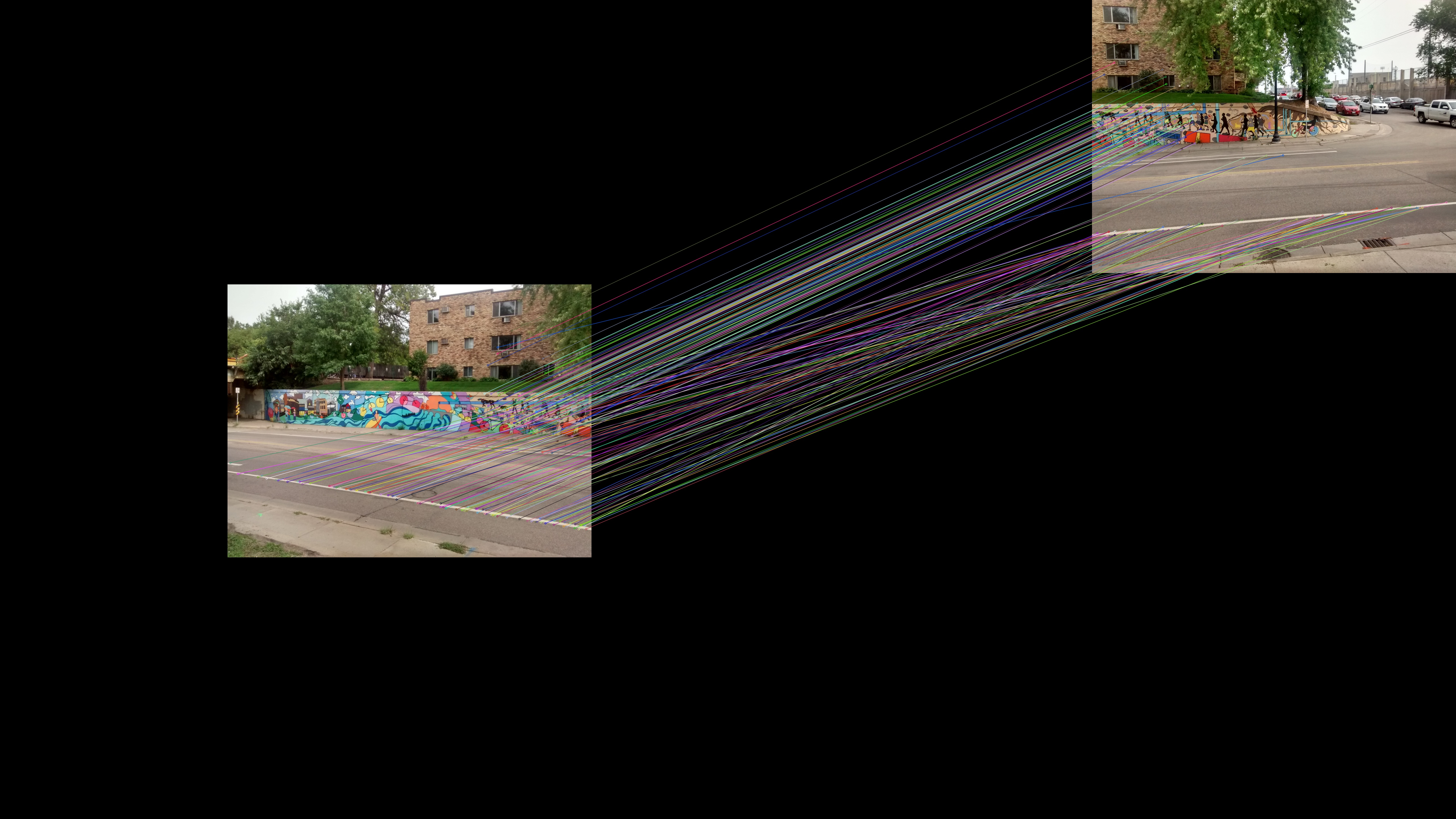(4.) Now since we have the best 200 SIFT matches between the images, we can find the Homography 1H2. As we know, we need only 4 matches at minimum to find the homography. But using more matches can improve the accuracy if we use RANSAC. RANSAC method try many different random subsets of the corresponding point pairs (of four pairs each), estimate the homography matrix using this subset and a simple least-square algorithm, and then compute the quality/goodness of the computed homography (which is the number of inliers for RANSAC or the median re-projection error for LMeDs). The best subset is then used to produce the initial estimate of the homography matrix and the mask of inliers/outliers.

// 1st image is the destination image and the 2nd image is the src image
std::vector<Point2f> dst_pts;                   //1st
std::vector<Point2f> source_pts;                //2nd

for (vector<DMatch>::iterator it = bestMatches.begin(); it != bestMatches.end(); ++it) {
//-- Get the keypoints from the good matches
dst_pts.push_back( keypoints_1[ it->queryIdx ].pt );
source_pts.push_back( keypoints_2[ it->trainIdx ].pt );
}

Mat H = findHomography( source_pts, dst_pts, CV_RANSAC );
cout << H << endl;


output:

[0.119035947337248, -0.04651626756941147, 1700.852494625838;
-0.5652916039380339, 0.9340629651977271, 1045.011078408947;
-0.0004251711674909509, 1.783961055570689e-05, 1]


(5.) Now we have the homography. We just need to apply the perspective warp on im_2 on a blank black canvas of the size of im_1. Let’s do it!

Mat wim_2;
warpPerspective(im_2, wim_2, H, im_1.size());


If you do a imshow on wim_2 i.e the warped image2 according to 1H2, on a bigger canvas, it will look like this: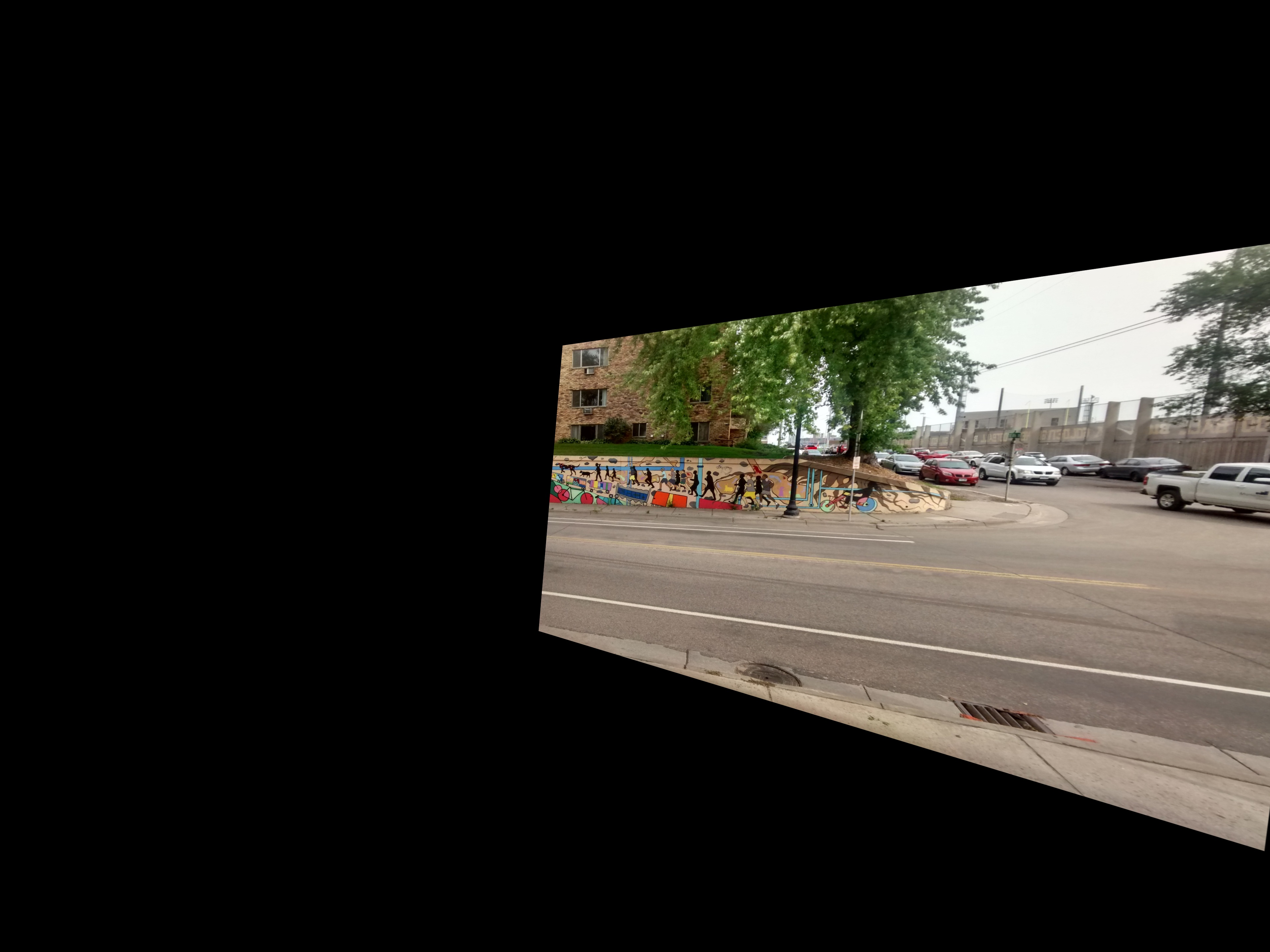(6.) Most of the things are done now. We just need to merge these two images together. A crude and simple method for this is: copy all those pixels from the wim_2 to im_1 which are black in im_1.

In code this will look like:

// We can do this since im_1 and wim_2 have the same size.
for (int i = 0; i < im_1.cols; i++)
for (int j = 0; j < im_1.rows; j++) {
Vec3b color_im1 = im_1.at<Vec3b>(Point(i, j));
Vec3b color_im2 = wim_2.at<Vec3b>(Point(i, j));
if (norm(color_im1) == 0)
im_1.at<Vec3b>(Point(i, j)) = color_im2;
}


Let’s see how im_1 looks now!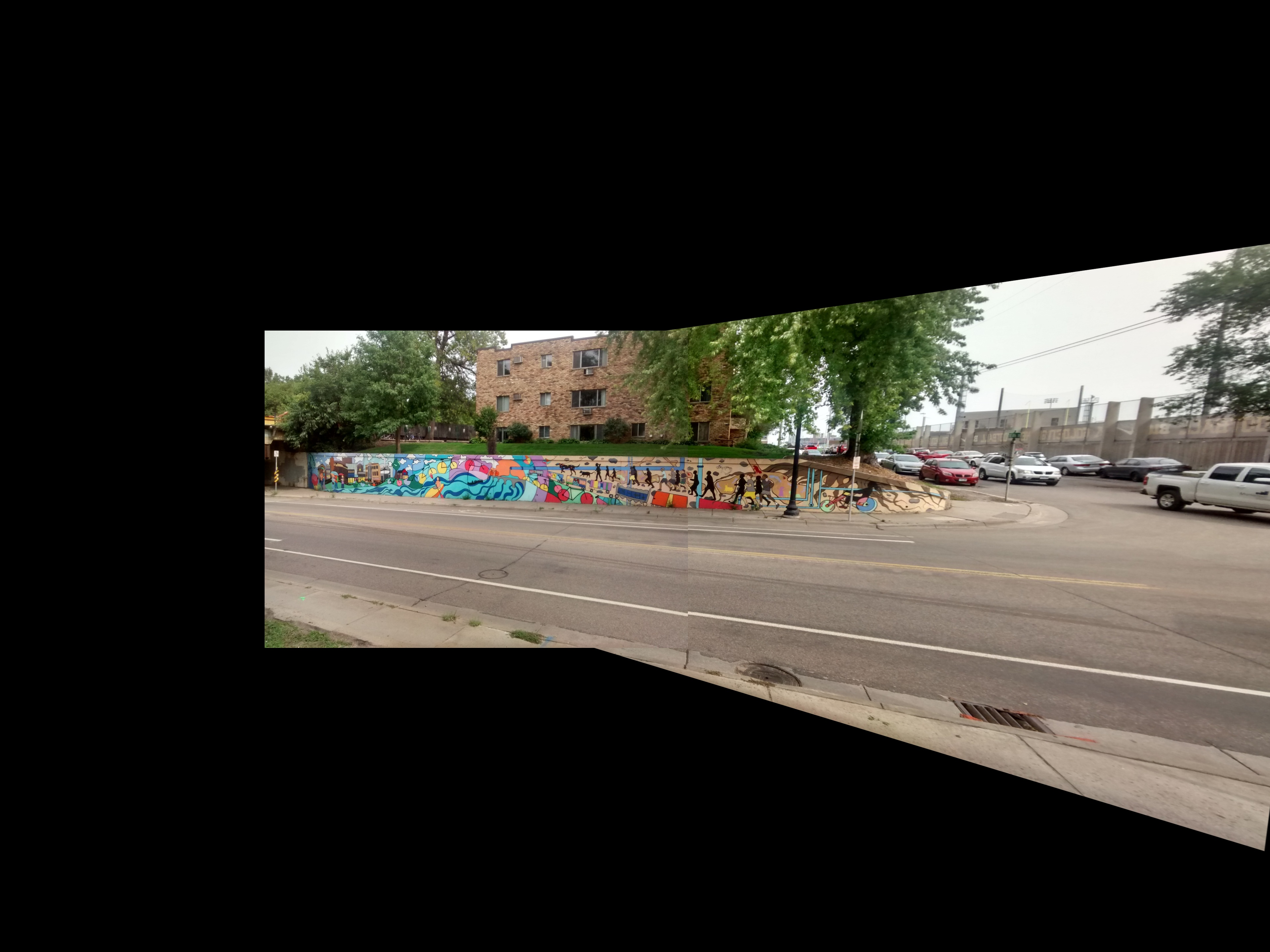TaDa! We have a basic Panorama out here! Now say you have many more images. Then it is better if you write two functions. One for shifting the dest image on the center of a bigger canvas and other for sticking im_2 on im_1 and returning a im_12. Now again run this function for sticking im_3 on im_12 to get im_123 … zzzz.

I hope you get the idea! If you didn’t get it … er er .. No problems! Here is the code: https://github.com/kislayabhi/blog_code/tree/master/OpenCV_pano